Publication date: 08/13/2020

## Limits

All data are treated as historical data when the number of historical rows is not specified in the launch window. See Launch the Model Driven Multivariate Control Chart Platform.

#### T2

The upper control limit (UCL) for historical data is based on the Beta distribution and defined as: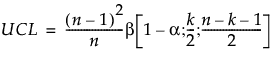where:

n = number of historical data observations

k = number of PCA or PLS components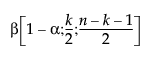= (1−α)th quantile of a Beta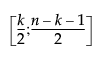distribution.

The UCL for current data is based on the F distribution and defined as: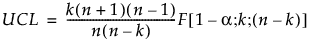where:

n = number of historical data observations

k = number of PCA or PLS components

F(1-α; k; n-k) = (1−α)th quantile of an F(k; n-k) distribution.

#### DModX

For PCA and PLS models, the UCL is based on the F distribution. The DModX UCL for PCA models is defined as: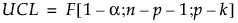where

n = number of historical data observations

k = number of PCA components

p = number of variables

F(1-α; n-p-1; p-k) = (1−α)th quantile of a F(n-p-1; p-k) distribution.

The DModX UCL for PLS models is defined as: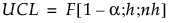where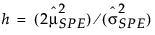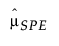= historical sample mean of SPE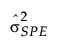= historical sample variance of SPE

n = number of historical data observations

F(1-α; h; nh) = (1−α)th quantile of an F(h; nh) distribution.

#### SPE

The SPE UCL for PCA models is defined as: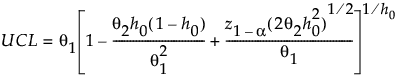where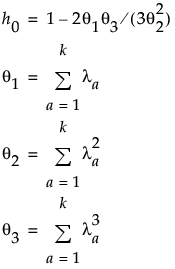λa = the ath eigenvalue

k = number of PCA components

z1−α = (1−α)th quantile of the standard normal distribution

For PLS models, the UCL is based on the chi-square distribution and defined as: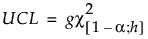where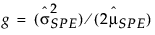= historical sample mean of SPE= historical sample variance of SPE

Χ2(1-α; h) = (1−α)th quantile of an Χ2(h) distribution

The g and h parameters are estimated by the method of moments. For more information about SPE control limits for PLS models, see Nomikos (1995).